# Andhra Pradesh SSC Board Question Paper for Class 10th Maths Paper 2 2019 In PDF

## AP SSC or 10th Class Question Paper Mathematics Paper 2 English Medium 2019 with Solutions – Free Download

Andhra Pradesh SSC (Class 10) Maths 2019 question paper 2 with solutions are available here in a downloadable pdf format and also in the text so that the students can easily obtain them. Along with the solutions, they can also get the Maths question paper 2 2019 class 10 SSC for reference. Students are able to access all the Andhra Pradesh board previous year Maths question papers here. AP 10th Class Mathematics Question Paper 2019 Paper 2 with Solutions can be downloaded easily and students can utilise them, practice and verify the answers provided by BYJU’S. Solving 2019 Maths question paper 2 for Class 10 will help the students to predict what type of questions will appear in the exam.

### QUESTION PAPER CODE 16E(A)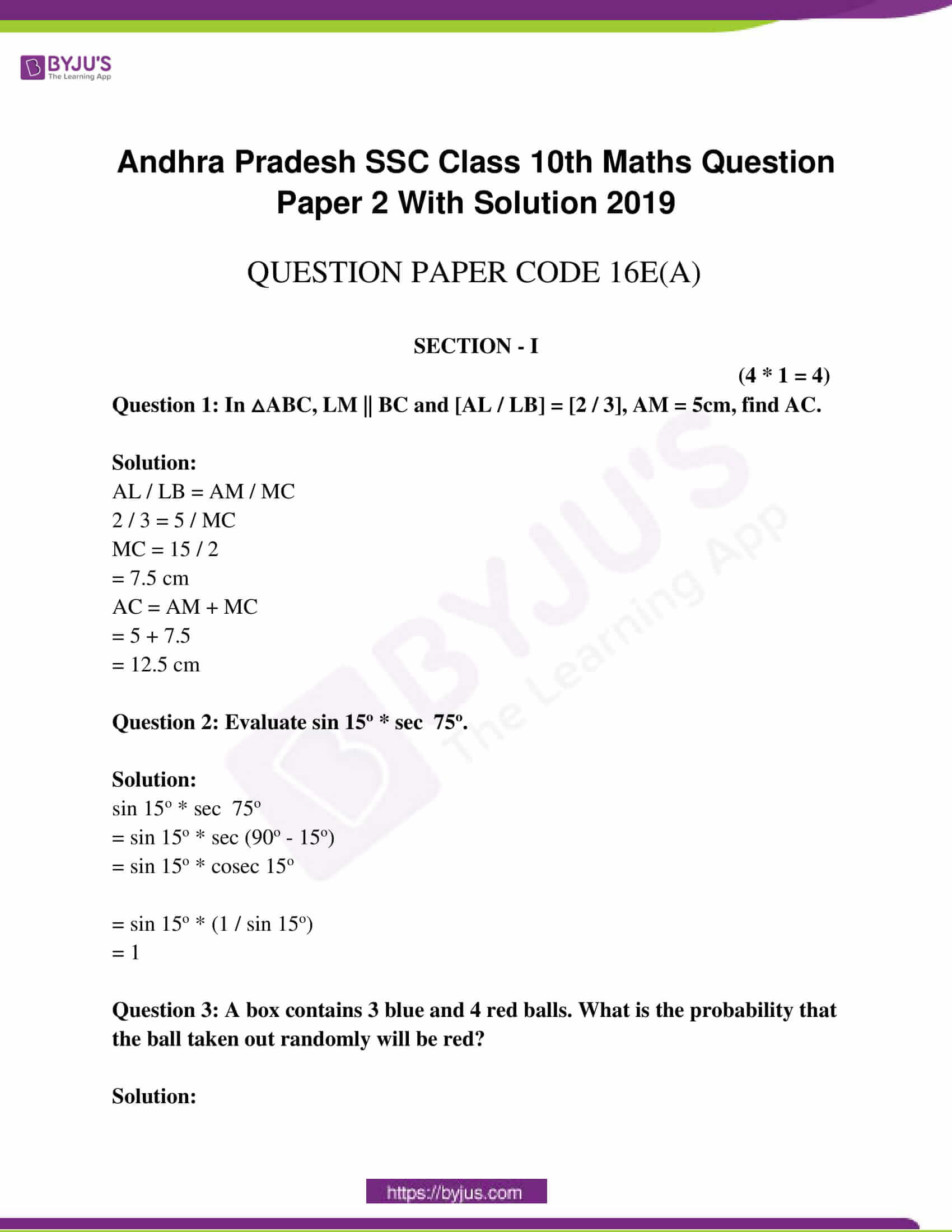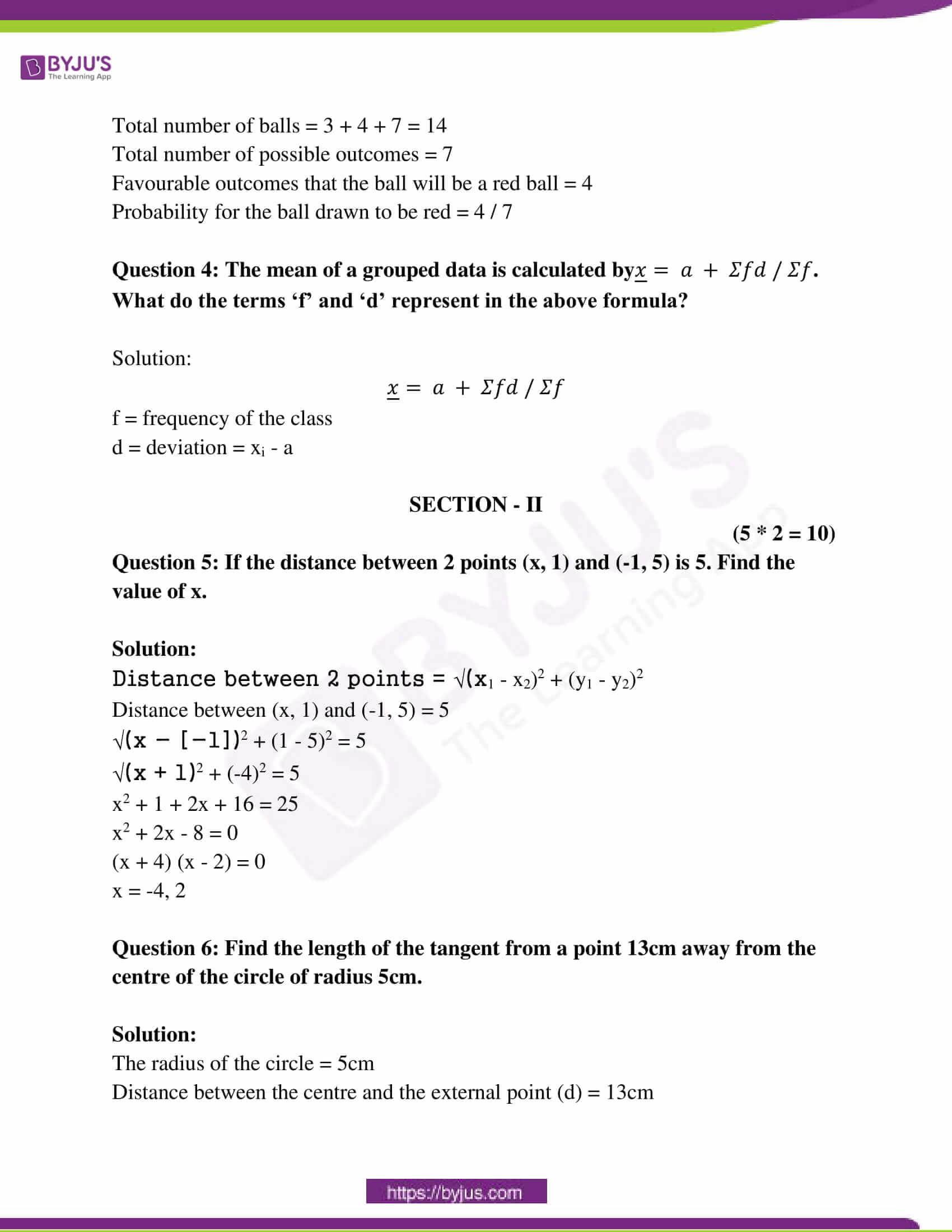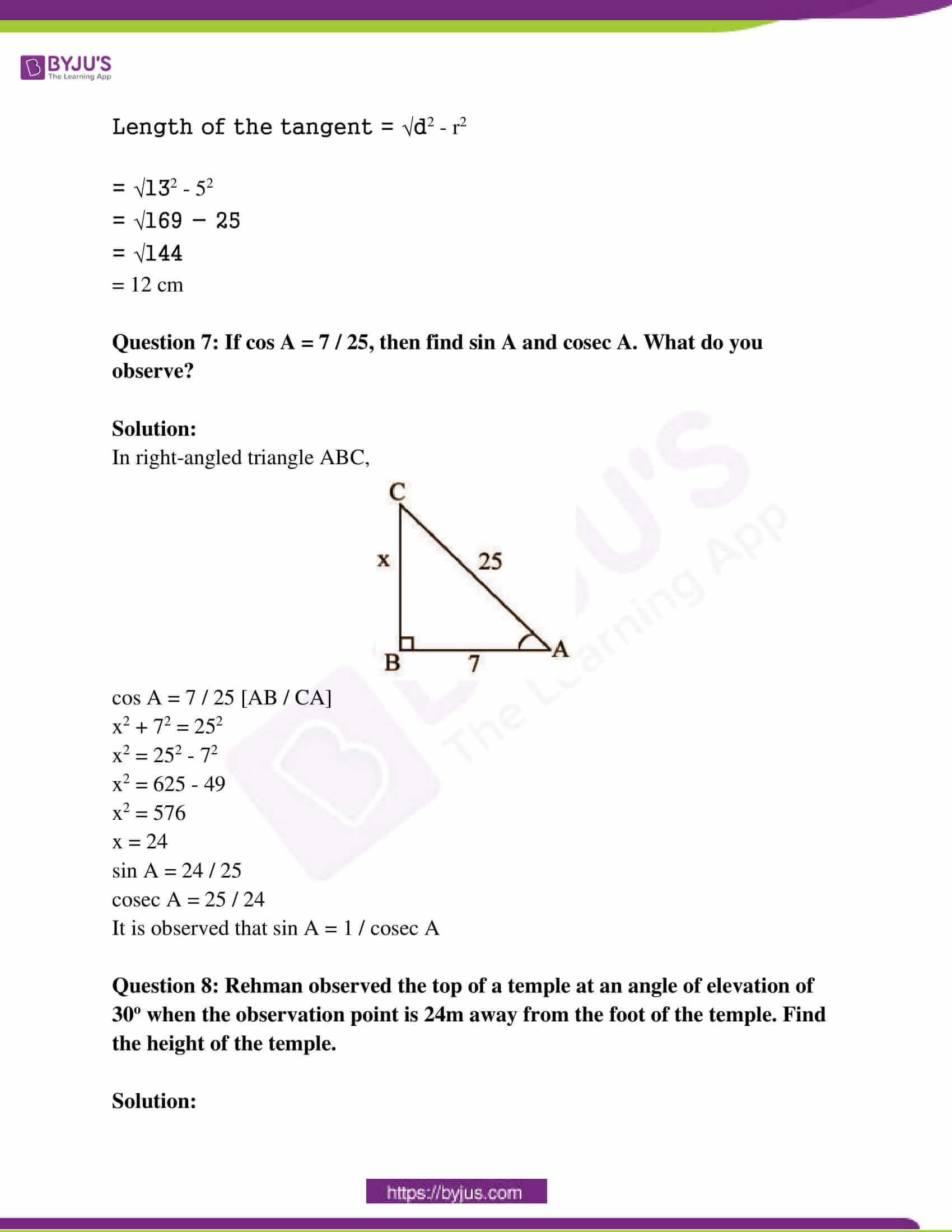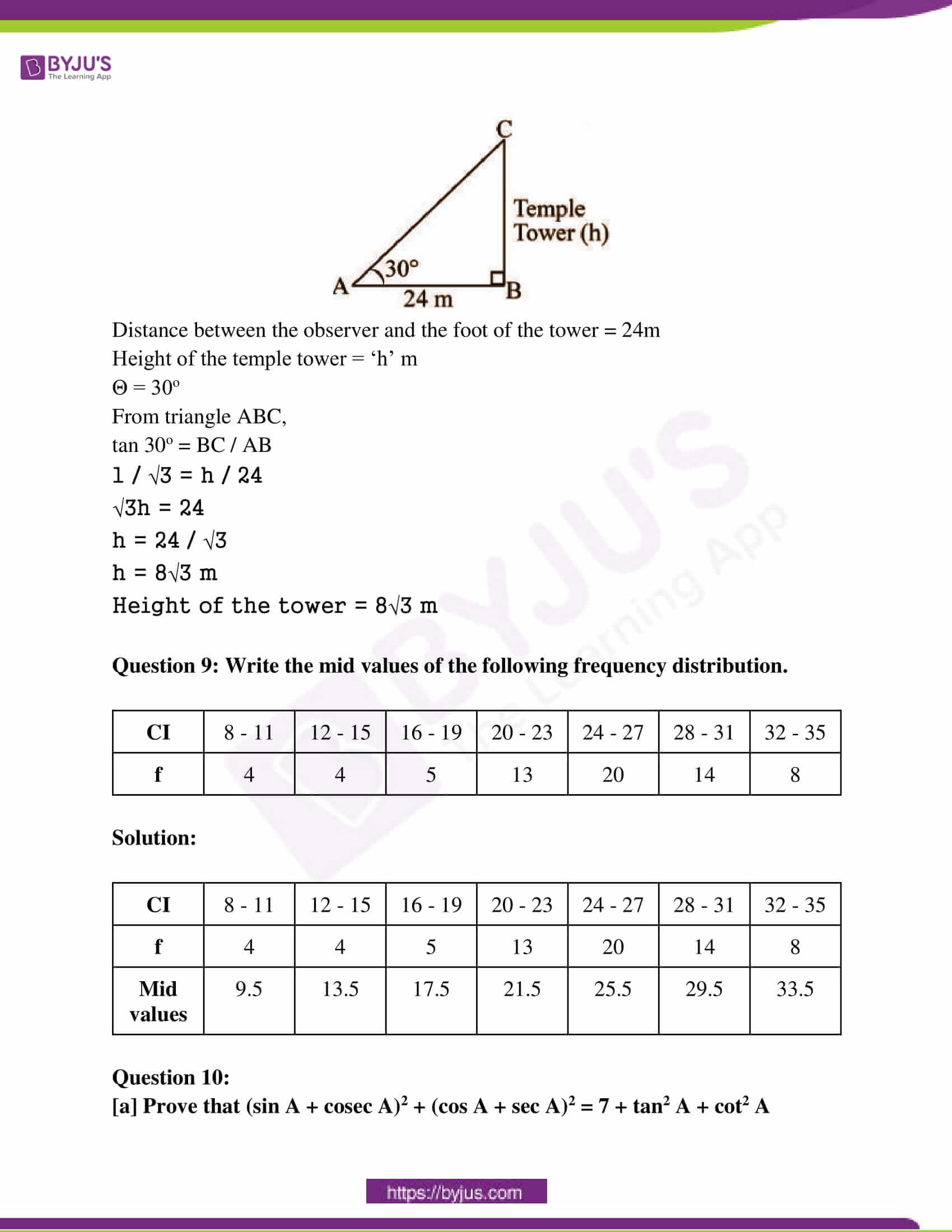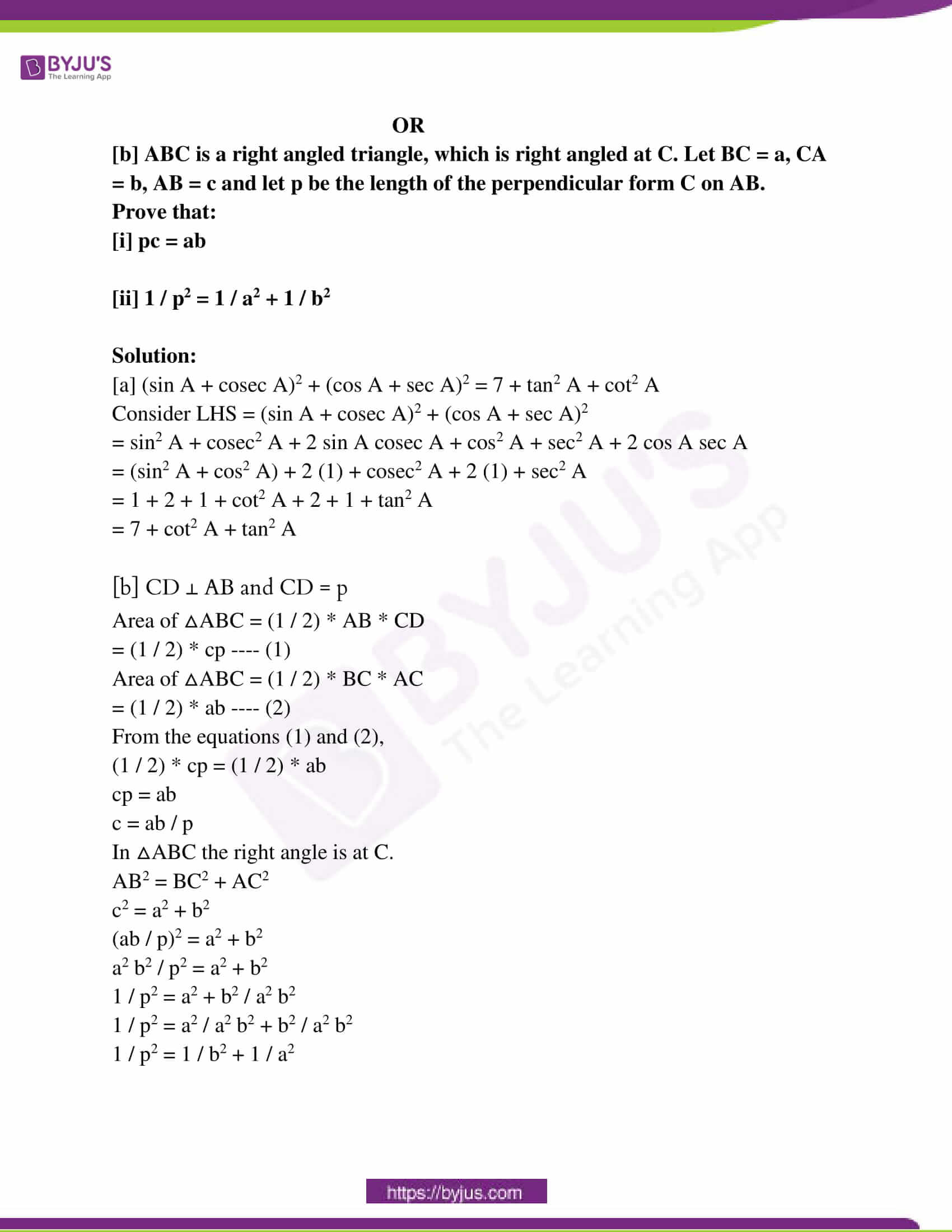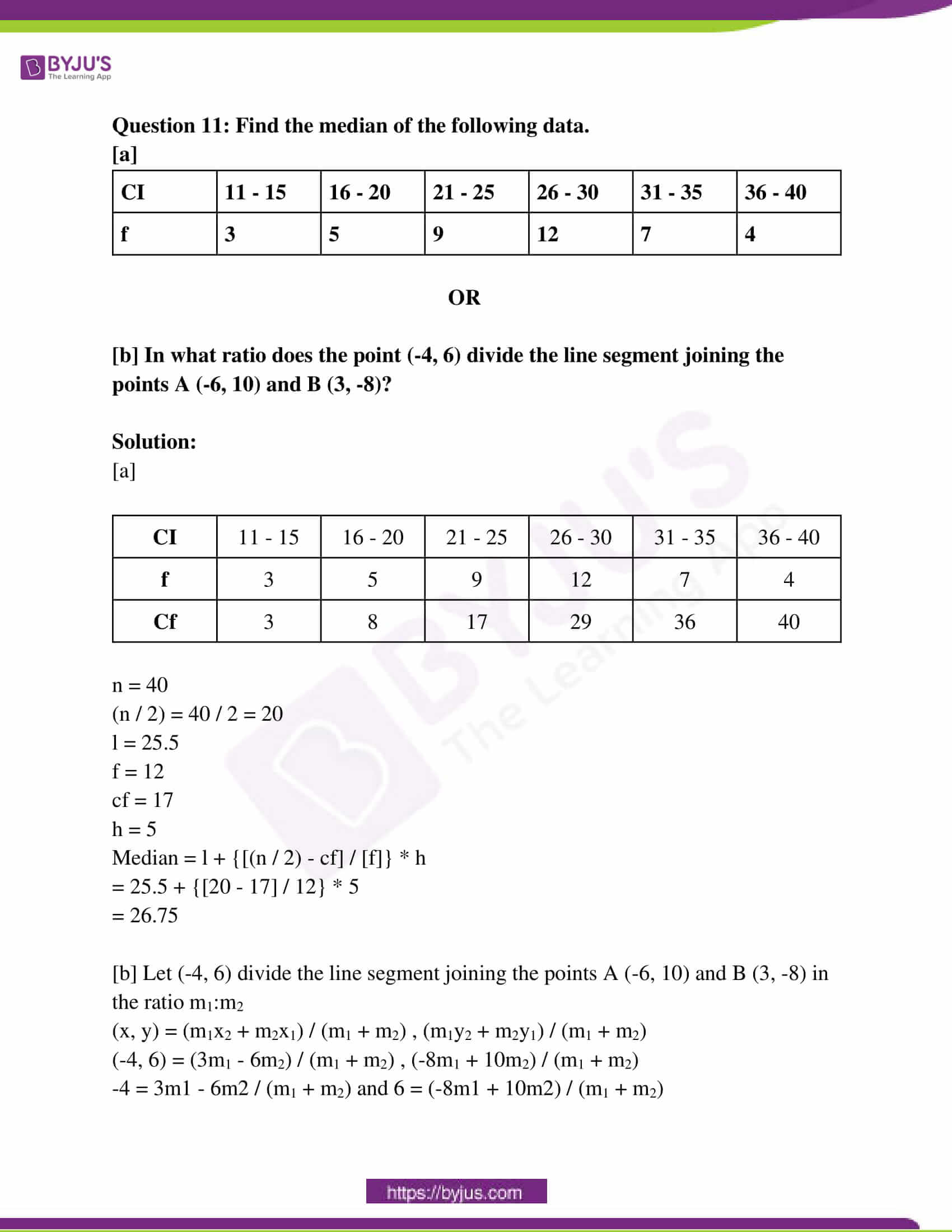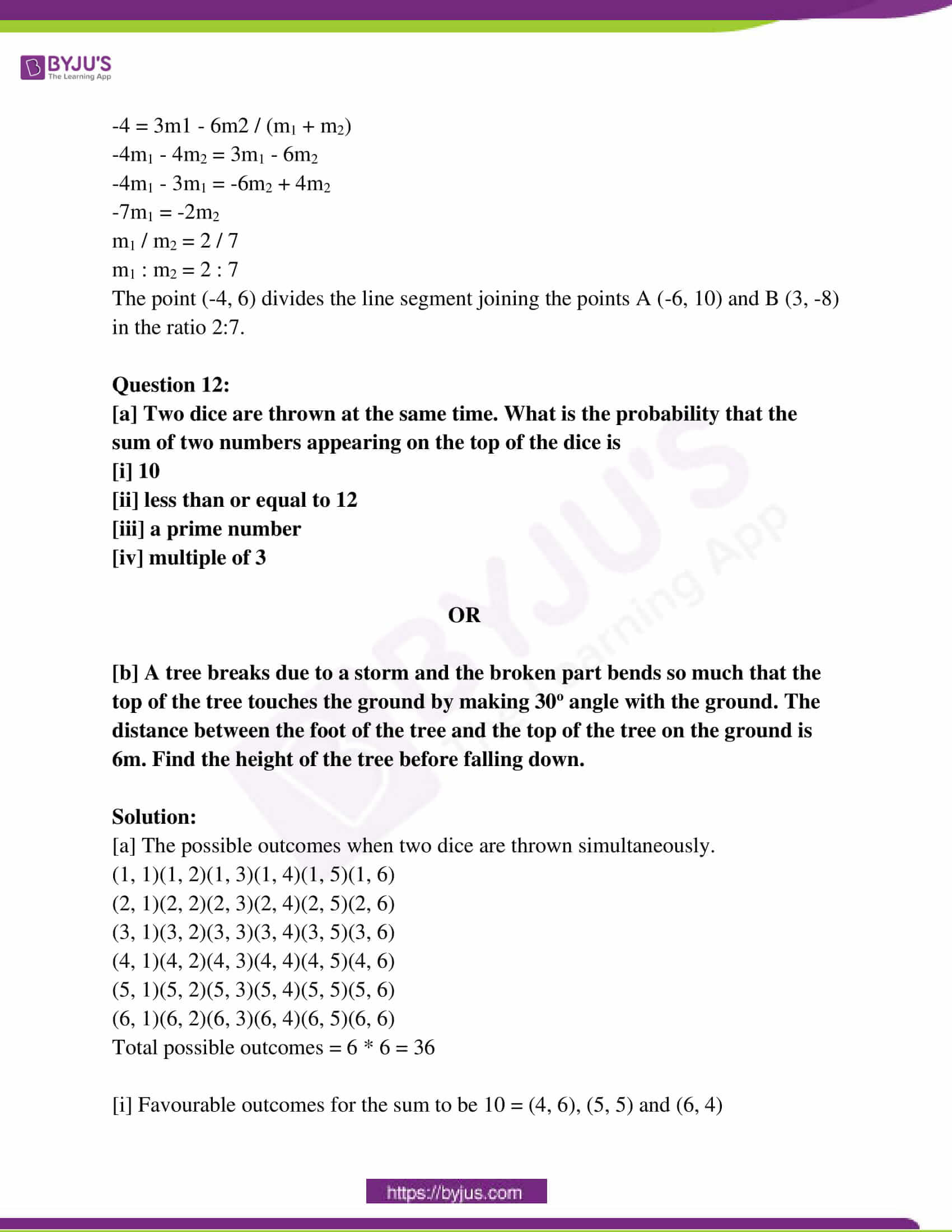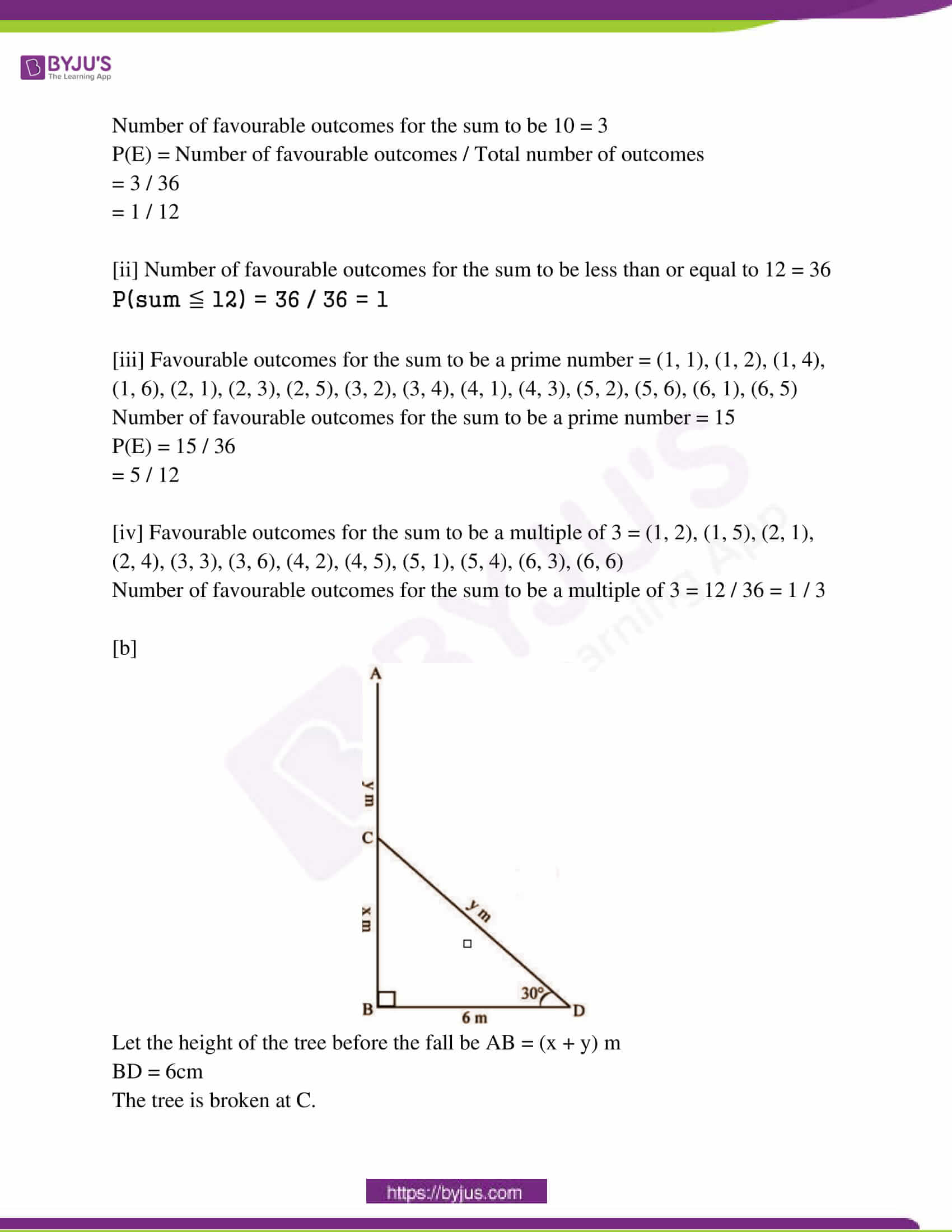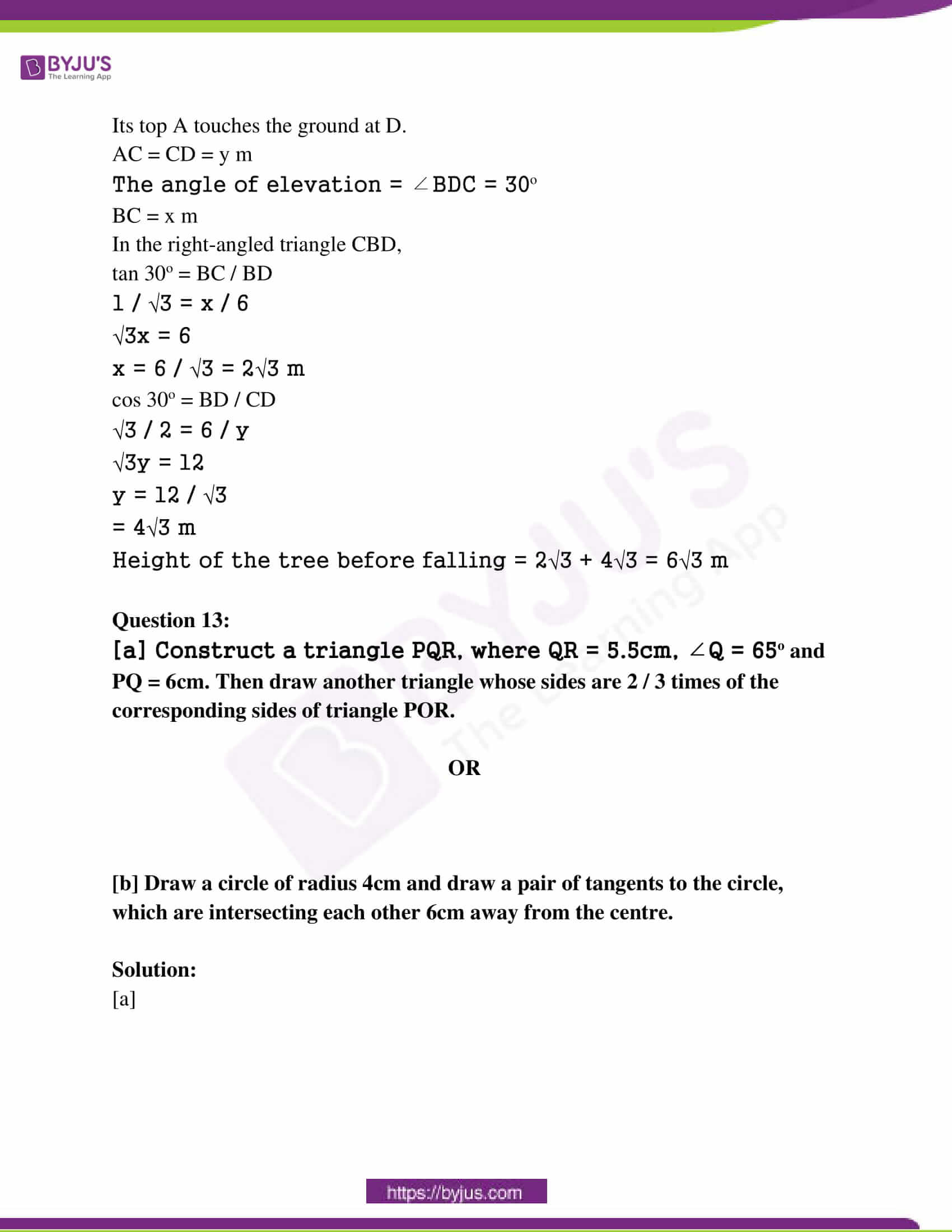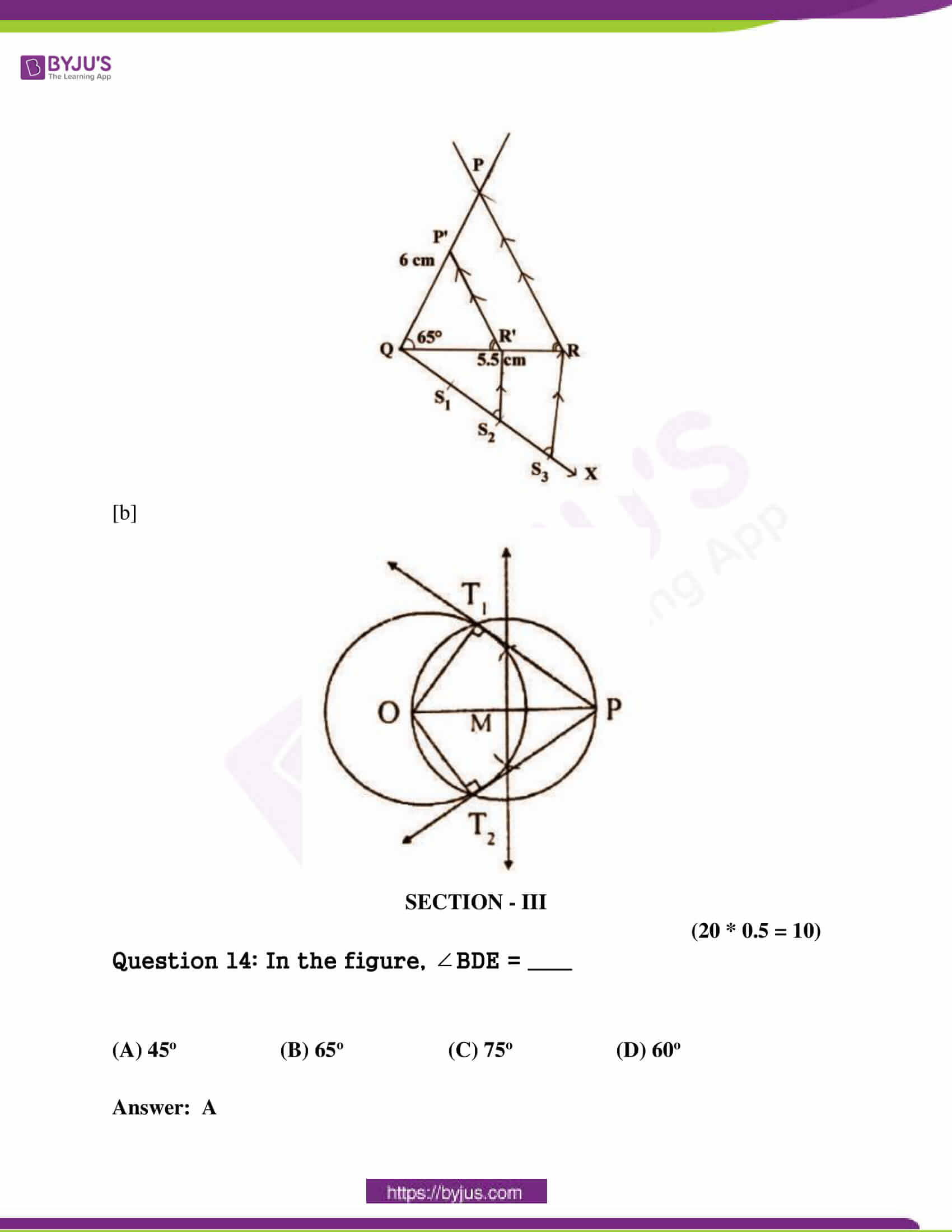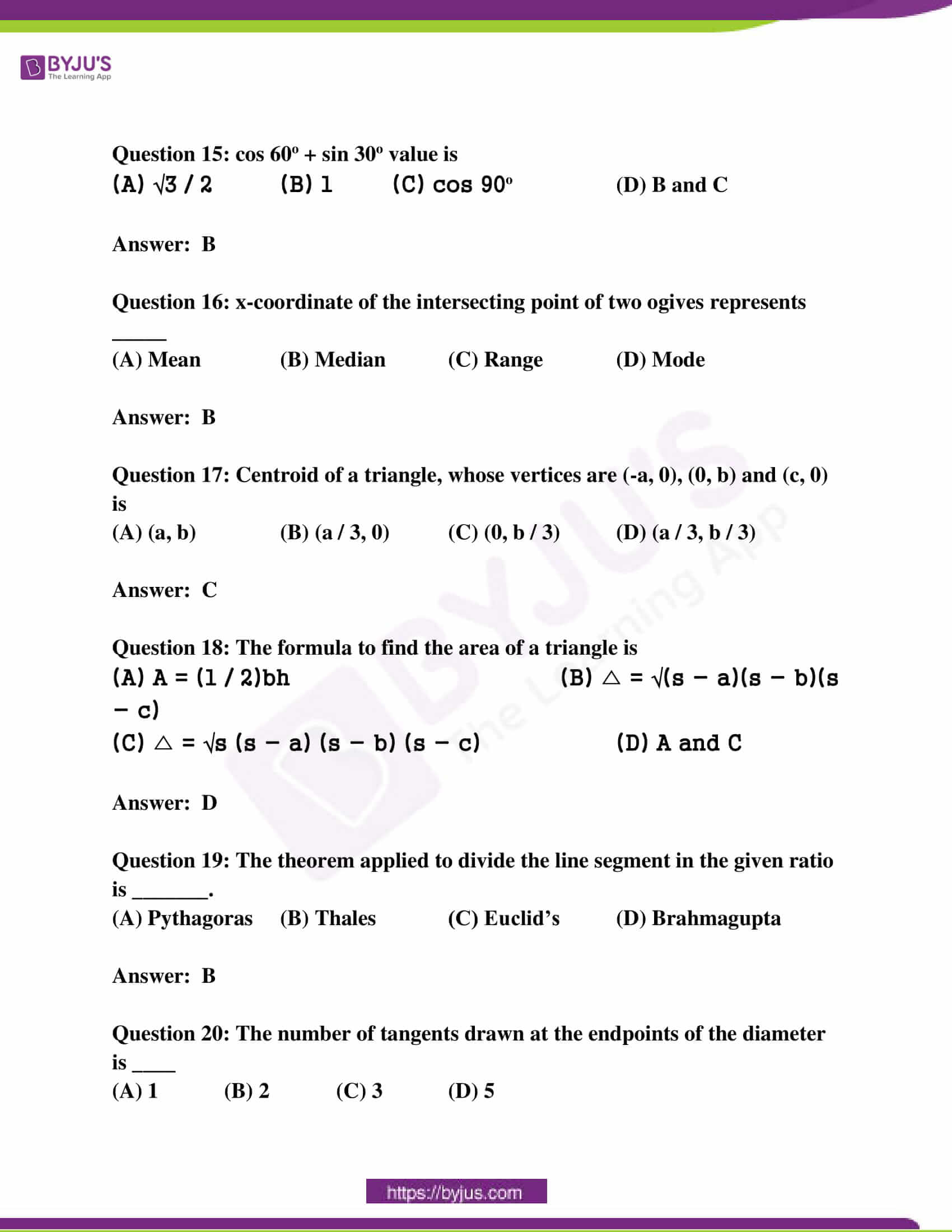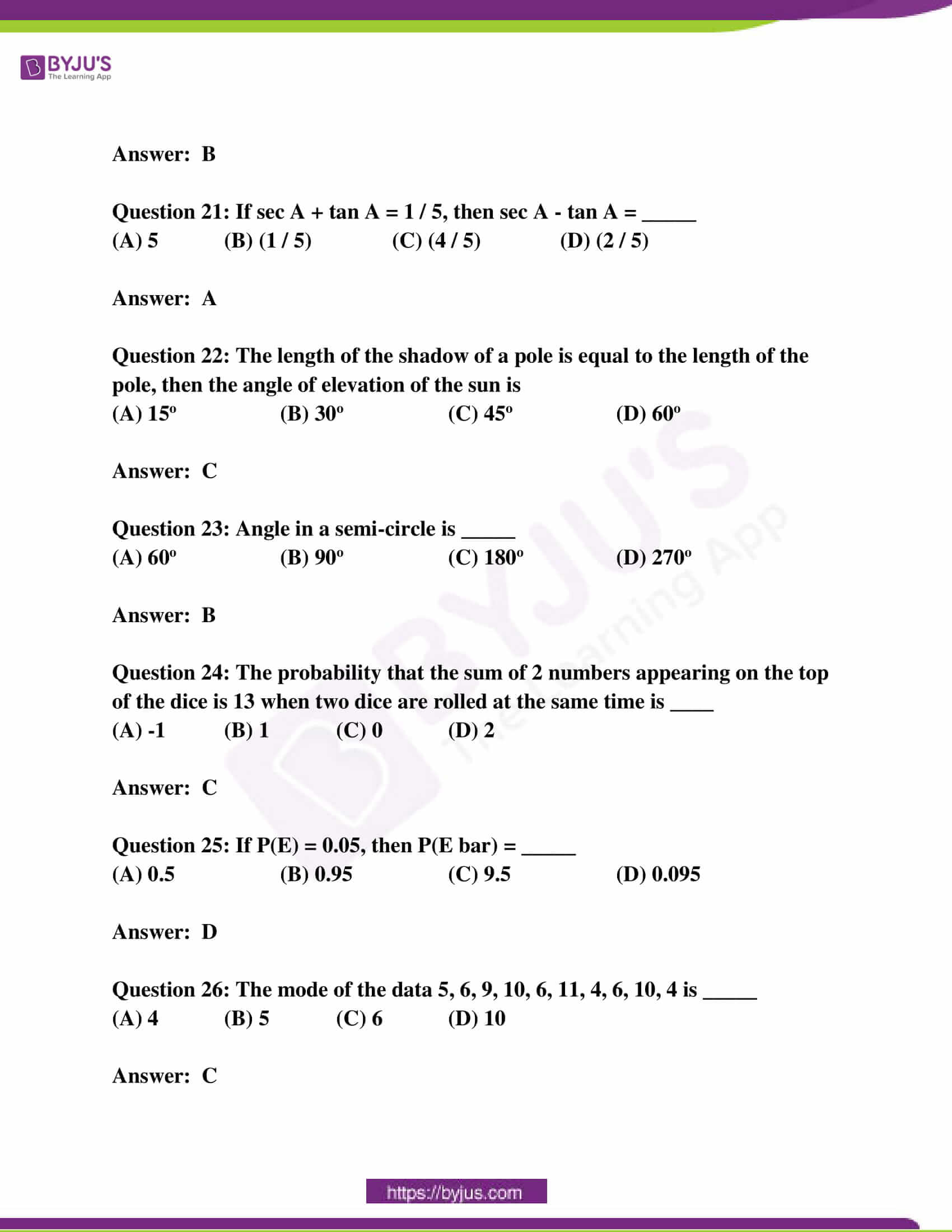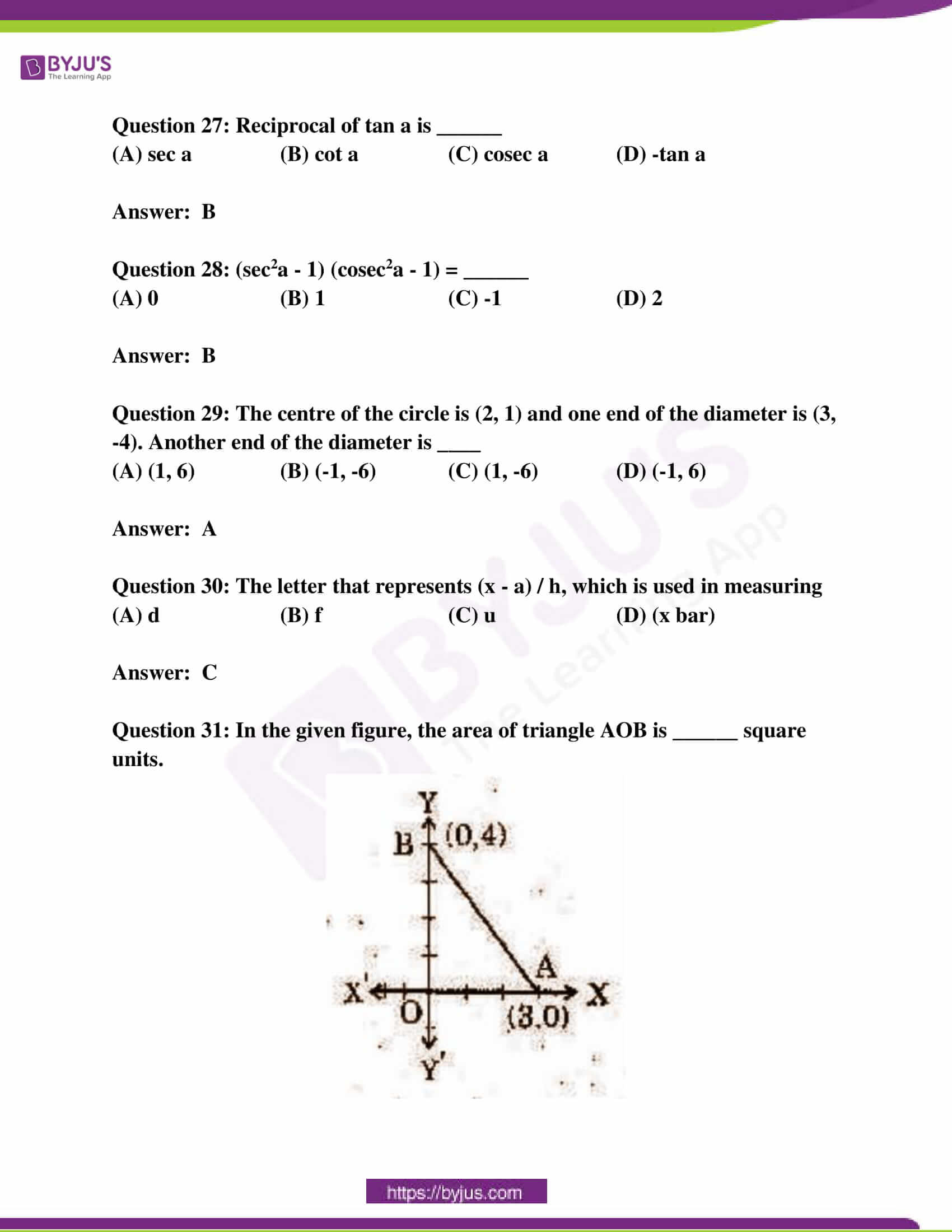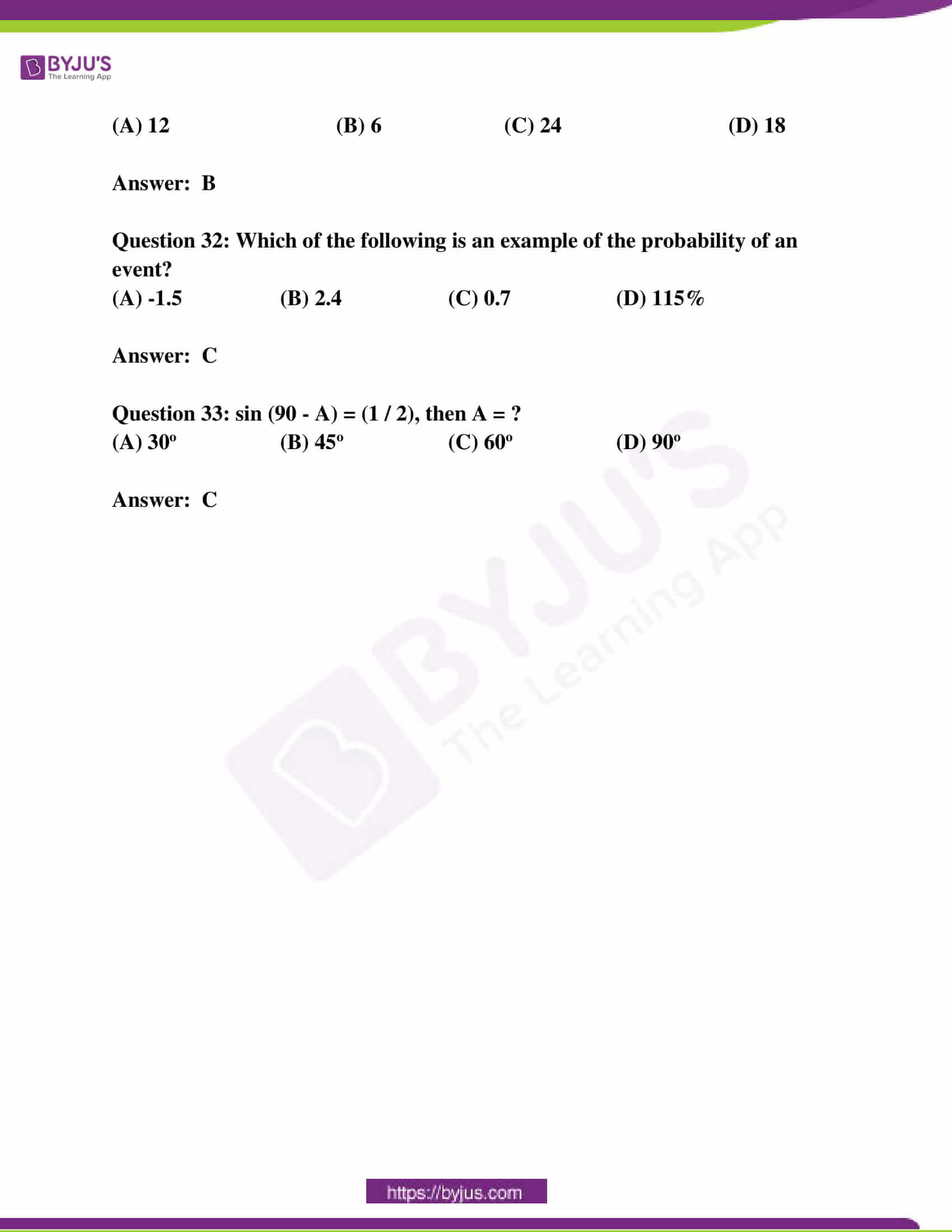SECTION – I

(4 * 1 = 4)

Question 1: In △ABC, LM || BC and [AL / LB] = [2 / 3], AM = 5cm, find AC.

Solution:

AL / LB = AM / MC

2 / 3 = 5 / MC

MC = 15 / 2

= 7.5 cm

AC = AM + MC

= 5 + 7.5

= 12.5 cm

Question 2: Evaluate sin 15o * sec 75o.

Solution:

sin 15o * sec 75o

= sin 15o * sec (90o – 15o)

= sin 15o * cosec 15o

= sin 15o * (1 / sin 15o)

= 1

Question 3: A box contains 3 blue and 4 red balls. What is the probability that the ball taken out randomly will be red?

Solution:

Total number of balls = 3 + 4 + 7 = 14

Total number of possible outcomes = 7

Favourable outcomes that the ball will be a red ball = 4

Probability for the ball drawn to be red = 4 / 7

Question 4: The mean of a grouped data is calculated by. What do the terms ‘f’ and ‘d’ represent in the above formula?

Solution:

f = frequency of the class

d = deviation = xi – a

SECTION – II

(5 * 2 = 10)

Question 5: If the distance between 2 points (x, 1) and (-1, 5) is 5. Find the value of x.

Solution:

Distance between 2 points = √(x1 – x2)2 + (y1 – y2)2

Distance between (x, 1) and (-1, 5) = 5

√(x – [-1])2 + (1 – 5)2 = 5

√(x + 1)2 + (-4)2 = 5

x2 + 1 + 2x + 16 = 25

x2 + 2x – 8 = 0

(x + 4) (x – 2) = 0

x = -4, 2

Question 6: Find the length of the tangent from a point 13cm away from the centre of the circle of radius 5cm.

Solution:

The radius of the circle = 5cm

Distance between the centre and the external point (d) = 13cm

Length of the tangent = √d2 – r2

= √132 – 52

= √169 – 25

= √144

= 12 cm

Question 7: If cos A = 7 / 25, then find sin A and cosec A. What do you observe?

Solution:

In right-angled triangle ABC,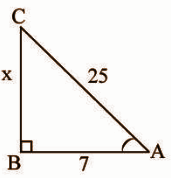cos A = 7 / 25 [AB / CA]

x2 + 72 = 252

x2 = 252 – 72

x2 = 625 – 49

x2 = 576

x = 24

sin A = 24 / 25

cosec A = 25 / 24

It is observed that sin A = 1 / cosec A

Question 8: Rehman observed the top of a temple at an angle of elevation of 30o when the observation point is 24m away from the foot of the temple. Find the height of the temple.

Solution: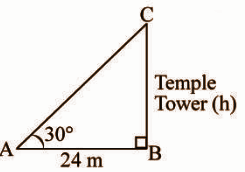Distance between the observer and the foot of the tower = 24m

Height of the temple tower = ‘h’ m

Θ = 30o

From triangle ABC,

tan 30o = BC / AB

1 / √3 = h / 24

√3h = 24

h = 24 / √3

h = 8√3 m

Height of the tower = 8√3 m

Question 9: Write the mid values of the following frequency distribution.

 CI 8 – 11 12 – 15 16 – 19 20 – 23 24 – 27 28 – 31 32 – 35 f 4 4 5 13 20 14 8

Solution:

 CI 8 – 11 12 – 15 16 – 19 20 – 23 24 – 27 28 – 31 32 – 35 f 4 4 5 13 20 14 8 Mid values 9.5 13.5 17.5 21.5 25.5 29.5 33.5

Question 10:

[a] Prove that (sin A + cosec A)2 + (cos A + sec A)2 = 7 + tan2 A + cot2 A

OR

[b] ABC is a right angled triangle, which is right angled at C. Let BC = a, CA = b, AB = c and let p be the length of the perpendicular form C on AB.

Prove that:

[i] pc = ab

[ii] 1 / p2 = 1 / a2 + 1 / b2

Solution:

[a] (sin A + cosec A)2 + (cos A + sec A)2 = 7 + tan2 A + cot2 A

Consider LHS = (sin A + cosec A)2 + (cos A + sec A)2

= sin2 A + cosec2 A + 2 sin A cosec A + cos2 A + sec2 A + 2 cos A sec A

= (sin2 A + cos2 A) + 2 (1) + cosec2 A + 2 (1) + sec2 A

= 1 + 2 + 1 + cot2 A + 2 + 1 + tan2 A

= 7 + cot2 A + tan2 A

[b] CD ⟂ AB and CD = p

Area of △ABC = (1 / 2) * AB * CD

= (1 / 2) * cp —- (1)

Area of △ABC = (1 / 2) * BC * AC

= (1 / 2) * ab —- (2)

From the equations (1) and (2),

(1 / 2) * cp = (1 / 2) * ab

cp = ab

c = ab / p

In △ABC the right angle is at C.

AB2 = BC2 + AC2

c2 = a2 + b2

(ab / p)2 = a2 + b2

a2 b2 / p2 = a2 + b2

1 / p2 = a2 + b2 / a2 b2

1 / p2 = a2 / a2 b2 + b2 / a2 b2

1 / p2 = 1 / b2 + 1 / a2

Question 11: Find the median of the following data.

[a]

 CI 11 – 15 16 – 20 21 – 25 26 – 30 31 – 35 36 – 40 f 3 5 9 12 7 4

OR

[b] In what ratio does the point (-4, 6) divide the line segment joining the points A (-6, 10) and B (3, -8)?

Solution:

[a]
 CI 11 – 15 16 – 20 21 – 25 26 – 30 31 – 35 36 – 40 f 3 5 9 12 7 4 Cf 3 8 17 29 36 40

n = 40

(n / 2) = 40 / 2 = 20

l = 25.5

f = 12

cf = 17

h = 5

Median = l + {[(n / 2) – cf] / [f]} * h

= 25.5 + {[20 – 17] / 12} * 5

= 26.75

[b] Let (-4, 6) divide the line segment joining the points A (-6, 10) and B (3, -8) in the ratio m1:m2

(x, y) = (m1x2 + m2x1) / (m1 + m2) , (m1y2 + m2y1) / (m1 + m2)

(-4, 6) = (3m1 – 6m2) / (m1 + m2) , (-8m1 + 10m2) / (m1 + m2)

-4 = 3m1 – 6m2 / (m1 + m2) and 6 = (-8m1 + 10m2) / (m1 + m2)

-4 = 3m1 – 6m2 / (m1 + m2)

-4m1 – 4m2 = 3m1 – 6m2

-4m1 – 3m1 = -6m2 + 4m2

-7m1 = -2m2

m1 / m2 = 2 / 7

m1 : m2 = 2 : 7

The point (-4, 6) divides the line segment joining the points A (-6, 10) and B (3, -8) in the ratio 2:7.

Question 12:

[a] Two dice are thrown at the same time. What is the probability that the sum of two numbers appearing on the top of the dice is

[i] 10

[ii] less than or equal to 12

[iii] a prime number

[iv] multiple of 3

OR

[b] A tree breaks due to a storm and the broken part bends so much that the top of the tree touches the ground by making 30o angle with the ground. The distance between the foot of the tree and the top of the tree on the ground is 6m. Find the height of the tree before falling down.

Solution:

[a] The possible outcomes when two dice are thrown simultaneously.

(1, 1)(1, 2)(1, 3)(1, 4)(1, 5)(1, 6)

(2, 1)(2, 2)(2, 3)(2, 4)(2, 5)(2, 6)

(3, 1)(3, 2)(3, 3)(3, 4)(3, 5)(3, 6)

(4, 1)(4, 2)(4, 3)(4, 4)(4, 5)(4, 6)

(5, 1)(5, 2)(5, 3)(5, 4)(5, 5)(5, 6)

(6, 1)(6, 2)(6, 3)(6, 4)(6, 5)(6, 6)

Total possible outcomes = 6 * 6 = 36

[i] Favourable outcomes for the sum to be 10 = (4, 6), (5, 5) and (6, 4)

Number of favourable outcomes for the sum to be 10 = 3

P(E) = Number of favourable outcomes / Total number of outcomes

= 3 / 36

= 1 / 12

[ii] Number of favourable outcomes for the sum to be less than or equal to 12 = 36

P(sum ≦ 12) = 36 / 36 = 1

[iii] Favourable outcomes for the sum to be a prime number = (1, 1), (1, 2), (1, 4), (1, 6), (2, 1), (2, 3), (2, 5), (3, 2), (3, 4), (4, 1), (4, 3), (5, 2), (5, 6), (6, 1), (6, 5)

Number of favourable outcomes for the sum to be a prime number = 15

P(E) = 15 / 36

= 5 / 12

[iv] Favourable outcomes for the sum to be a multiple of 3 = (1, 2), (1, 5), (2, 1), (2, 4), (3, 3), (3, 6), (4, 2), (4, 5), (5, 1), (5, 4), (6, 3), (6, 6)

Number of favourable outcomes for the sum to be a multiple of 3 = 12 / 36 = 1 / 3

[b]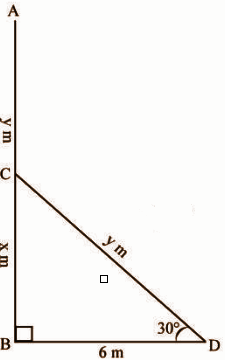Let the height of the tree before the fall be AB = (x + y) m

BD = 6cm

The tree is broken at C.

Its top A touches the ground at D.

AC = CD = y m

The angle of elevation = ∠BDC = 30o

BC = x m

In the right-angled triangle CBD,

tan 30o = BC / BD

1 / √3 = x / 6

√3x = 6

x = 6 / √3 = 2√3 m

cos 30o = BD / CD

√3 / 2 = 6 / y

√3y = 12

y = 12 / √3

= 4√3 m

Height of the tree before falling = 2√3 + 4√3 = 6√3 m

Question 13:

[a] Construct a triangle PQR, where QR = 5.5cm, ∠Q = 65o and PQ = 6cm. Then draw another triangle whose sides are 2 / 3 times of the corresponding sides of triangle POR.

OR

[b] Draw a circle of radius 4cm and draw a pair of tangents to the circle, which are intersecting each other 6cm away from the centre.

Solution:

[a]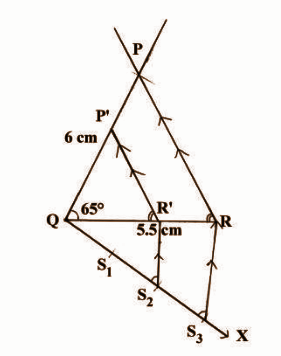[b]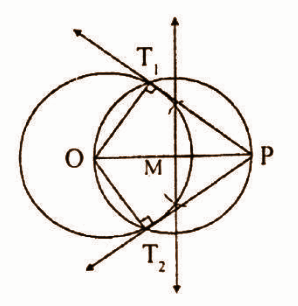SECTION – III

(20 * 0.5 = 10)

Question 14: In the figure, ∠BDE = ____

(A) 45o (B) 65o (C) 75o (D) 60o

Question 15: cos 60o + sin 30o value is

(A) √3 / 2 (B) 1 (C) cos 90o (D) B and C

Question 16: x-coordinate of the intersecting point of two ogives represents _____

(A) Mean (B) Median (C) Range (D) Mode

Question 17: Centroid of a triangle, whose vertices are (-a, 0), (0, b) and (c, 0) is

(A) (a, b) (B) (a / 3, 0) (C) (0, b / 3) (D) (a / 3, b / 3)

Question 18: The formula to find the area of a triangle is

(A) A = (1 / 2)bh (B) △ = √(s – a)(s – b)(s – c)

(C) △ = √s (s – a) (s – b) (s – c) (D) A and C

Question 19: The theorem applied to divide the line segment in the given ratio is _______.

(A) Pythagoras (B) Thales (C) Euclid’s (D) Brahmagupta

Question 20: The number of tangents drawn at the endpoints of the diameter is ____

(A) 1 (B) 2 (C) 3 (D) 5

Question 21: If sec A + tan A = 1 / 5, then sec A – tan A = _____

(A) 5 (B) (1 / 5) (C) (4 / 5) (D) (2 / 5)

Question 22: The length of the shadow of a pole is equal to the length of the pole, then the angle of elevation of the sun is

(A) 15o (B) 30o (C) 45o (D) 60o

Question 23: Angle in a semi-circle is _____

(A) 60o (B) 90o (C) 180o (D) 270o

Question 24: The probability that the sum of 2 numbers appearing on the top of the dice is 13 when two dice are rolled at the same time is ____

(A) -1 (B) 1 (C) 0 (D) 2

Question 25: If P(E) = 0.05, then P(E bar) = _____

(A) 0.5 (B) 0.95 (C) 9.5 (D) 0.095

Question 26: The mode of the data 5, 6, 9, 10, 6, 11, 4, 6, 10, 4 is _____

(A) 4 (B) 5 (C) 6 (D) 10

Question 27: Reciprocal of tan a is ______

(A) sec a (B) cot a (C) cosec a (D) -tan a

Question 28: (sec2a – 1) (cosec2a – 1) = ______

(A) 0 (B) 1 (C) -1 (D) 2

Question 29: The centre of the circle is (2, 1) and one end of the diameter is (3, -4). Another end of the diameter is ____

(A) (1, 6) (B) (-1, -6) (C) (1, -6) (D) (-1, 6)

Question 30: The letter that represents (x – a) / h, which is used in measuring

(A) d (B) f (C) u (D) (x bar)

Question 31: In the given figure, the area of triangle AOB is ______ square units.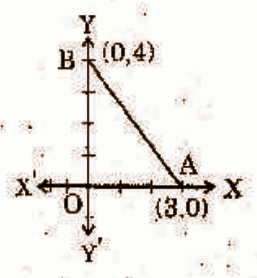(A) 12 (B) 6 (C) 24 (D) 18

Question 32: Which of the following is an example of the probability of an event?

(A) -1.5 (B) 2.4 (C) 0.7 (D) 115%